<img src="https://trc.taboola.com/1278851/log/3/unip?en=page_view" width="0" height="0" style="display:none">

# Data Analysis using Dynamic Array formulasAmin Mohammed
September 17, 2020

# How to create reports using dynamic array formulas in Planning Analytics TM1

In our previous blog (https://blog.octanesolutions.com.au/what-are-dynamic-array-formulas-and-why-you-should-use-them), we discussed about Dynamic Array formulas and highlighted the key reasons and advantages to start using DA formulas.

In this blog, we will try to create a few intuitive reports based on custom reports built on PAfE. The data set we will be using is shows the employee details in “Employee” cube with the following dimensionality:

 Dimension Measure Year Department Version Name/Desc Sr.No Current Salary Organisation Joining Date Measure

Below is the screenshot of my PA data that I will be using for this blog: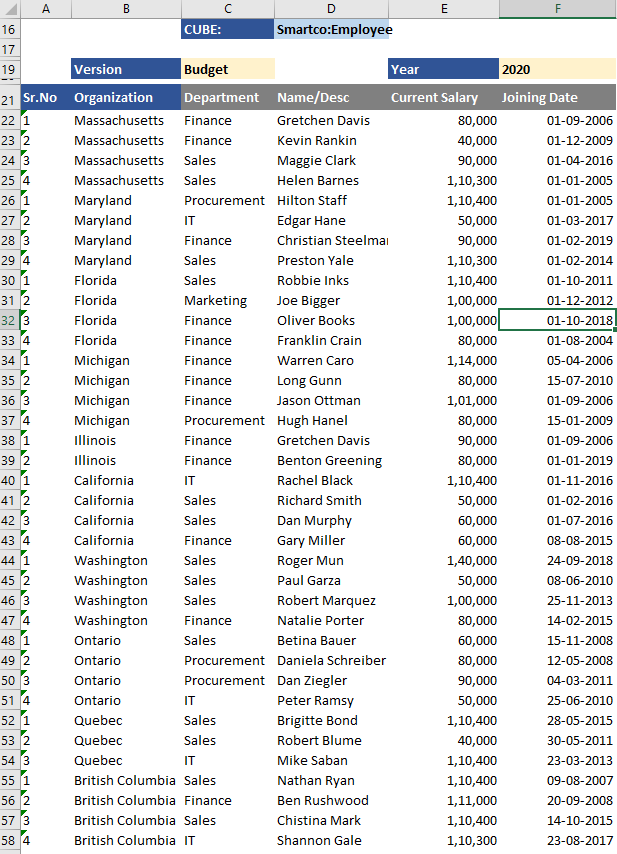For ease of formula entry, I’ve created a named range for column B to F.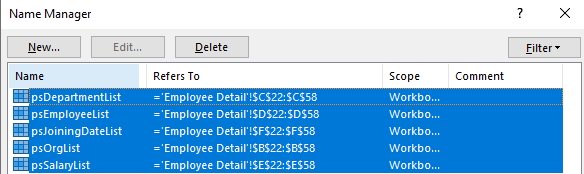Now that we’ve set the base, lets start off with generating some useful insights with our dataset.

1. Get the employees with top/bottom 3 salaries
2. Sum data based on date range
3. Create searchable drop down list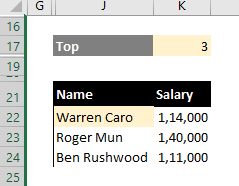Formula in cell J22 is as below: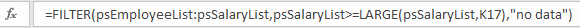I will try to breakdown the formula to explain in simple language:

We used Filter function which is a DA formula. The Excel FILTER function filters a range of data based on supplied criteria, and extracts matching records. It works in a similar way to VLOOKUP except that VLOOKUP returns a single value, whereas Filter returns one or more values that qualify a criteria. Filter takes three arguments; Array, Include and If_Empty. We passed the employee and salary list as the array in our formula and for inclusion we used a LARGE function (that returns the x largest value in an array where x is a number) and compared it with all the salaries using greater than or equal to operator.

With this criteria, the array is filtered to those employees whose salary is greater than or equal to the 3rd most largest salary.

Similarly, if you wish to filter the employees by 3 lowest salaries. Use the below formula to achieve the same: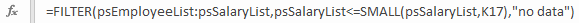A very common analysis that is done based on date range is summarising or calculating the average of data between start and end date. So lets see how we can achieve this using the DA formula. The scenario is, the analyst wants to see what is the sum of the salaries paid for all the periods between Jan 2019 till Dec 2019.

Lets first get the list using the Filter function and once we’ve the data, it is very easy to just summarise it.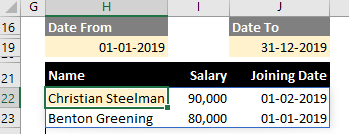Formula in cell H22 is as below: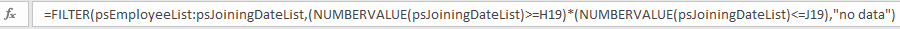The concept is similar to the previous one where we’re getting a list of employees with their salaries and joining dates, based on a set condition. Here we’re using AND condition to filter the data based on two date ranges, where joining date of employees is greater than or equal to Date From and less than or equal to To Date. We had to use the NUMBERVALUE function to convert the date that is stored as string data in Planning Analytics to numeric value for doing logical comparison.

Now that we know we can apply the same condition within the Filter function that only returns the Salary and wrap it up inside the SUM function to summarise the salaries.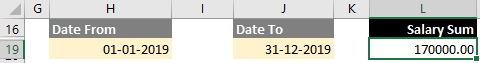Formula in cell L19: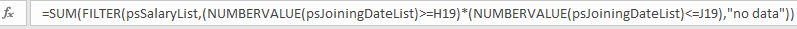In PAfE, a SUBNM is used to search and select the elements in a dimension. However, there is currently no provision to filter the list of elements in SUBNM list to only show selected elements basis that matches the text, let alone wild card search. One of the cool things we can do with DA formulas is to be able to create a searchable drop down list.

Lets create a searchable drop down list for the Department now and see how it works.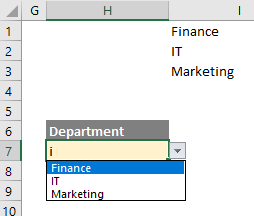In the screenshot above, I’ve entered letter i in cell H7 which is a Data Validation list in Excel and the drop down lists all the departments which have letter i in it. The actual formula is written in cell I1 and that cell is referenced in the Source field of Data Validation.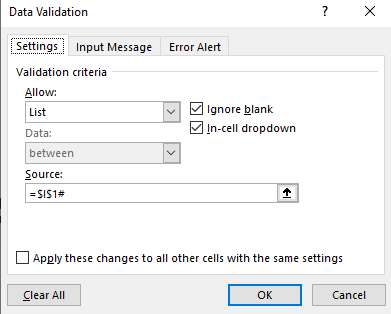I’ve used a Hash(#) character in the source to refer to an entire spill range that the formula in I1 returns.

Formula in cell I1: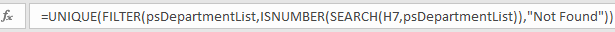I’ve wrapped a Filter function within a UNIQUE function that is another DA function that returns a unique list of values within an array. The Filter function uses SEARCH function to return a value if a match is found which is then wrapped inside ISNUMBER to return a Boolean value.

Note:: While the example uses custom report, the same named ranges can very well be created in Dynamic report using OFFSET function to do the same so this analysis is not just restricted to sliced report but also Dynamic aka Active Form report.

So these are just a few of the super easy and on the fly analysis we can do using DA functions to start with that can take the reporting capabilities of PAfE to a whole new level.Amin Mohammed